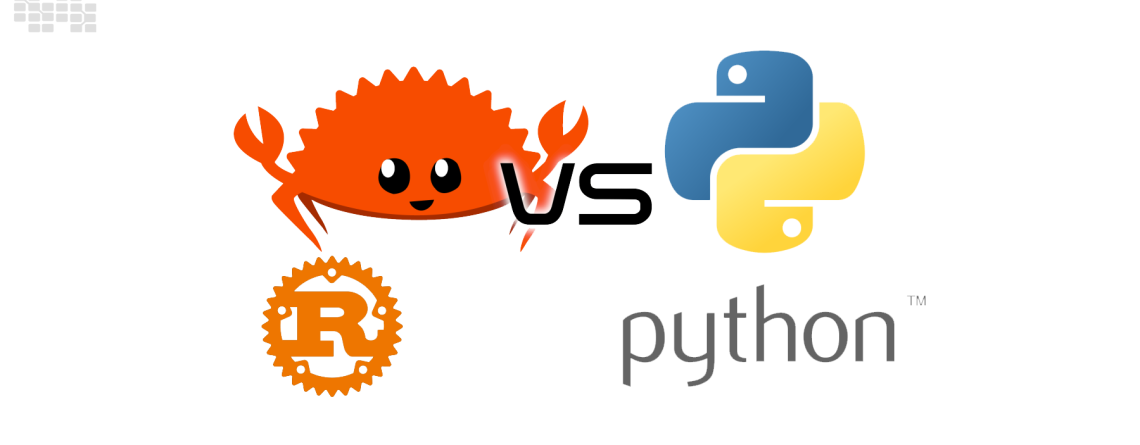# Rust vs. Python: The Battle for Deep Learning Dominance## Rust vs. Python: The Battle for Deep Learning Dominance

Artificial intelligence has undergone a revolution because to deep learning, which allows machines to comprehend, analyze, and even generate complicated data patterns. The selection of a programming language for deep learning implementation is becoming more and more important as it gains pace. In this blog article, we’ll examine the race for supremacy in deep learning between two strong competitors: Python and Rust.

## Python: The King of Data Science

Python has long been the de facto language for data science and machine learning. Its dominance is evident in the plethora of libraries and frameworks, such as TensorFlow, PyTorch, and Keras, specifically designed for deep learning. Let’s start by exploring a simple Python example for training a basic neural network using TensorFlow:

```import tensorflow as tf
from tensorflow import keras

# Create a basic neural network model
model = keras.Sequential([
keras.layers.Dense(64, activation='relu', input_shape=(784,)),
keras.layers.Dropout(0.5),
keras.layers.Dense(10, activation='softmax')
])

# Compile the model
loss='sparse_categorical_crossentropy',
metrics=['accuracy'])

mnist = keras.datasets.mnist
(train_images, train_labels), (test_images, test_labels) = mnist.load_data()
train_images = train_images.reshape((-1, 784)).astype('float32') / 255.0
test_images = test_images.reshape((-1, 784)).astype('float32') / 255.0

# Train the model
model.fit(train_images, train_labels, epochs=5)

# Evaluate the model
test_loss, test_acc = model.evaluate(test_images, test_labels)
print(f'Test accuracy: {test_acc}')```

Python’s user-friendly syntax and extensive libraries make it an attractive choice for deep learning. However, its performance can be a concern, especially when dealing with large datasets or complex models.

## Rust: The New Challenger

Rust, known for its emphasis on performance and memory safety, has entered the deep learning arena as a worthy challenger. Although Rust’s deep learning ecosystem is not as mature as Python’s, its unique strengths cannot be ignored. Let’s examine a Rust example for training a similar neural network using the `tch` (Torch-rs) crate:

```extern crate tch;
use tch::{nn, nn::Module, nn::OptimizerConfig, nn::OptimizerConfigBuilder, nn::VarStore, nn::ModuleT};
use tch::nn::LinearConfig;
use tch::nn::ModuleConfig;
use tch::vision::mnist;
use tch::Tensor;
use tch::nn::Loss;

struct Net {
fc1: nn::Linear,
fc2: nn::Linear,
}

impl Net {
fn new(vs: &nn::Path) -> Net {
let fc1 = nn::linear(vs, 784, 64, Default::default());
let fc2 = nn::linear(vs, 64, 10, Default::default());
Net { fc1, fc2 }
}
}

impl nn::Module for Net {
fn forward(&self, xs: &Tensor) -> Tensor {
xs.view([-1, 784])
.forward(&self.fc1)
.relu()
.forward(&self.fc2)
}
}

fn main() {
let vs = tch::nn::VarStore::new(tch::Device::Cuda(0)).unwrap();
let net = Net::new(&vs.root());

let mut opt = nn::Adam::default().build(&vs, 1e-3).unwrap();

for epoch in 1..=5 {
for (bimages, blabels) in train.iter() {
let loss_value = net
.forward(&bimages)
.cross_entropy_for_logits(&blabels);
opt.backward_step(&loss_value);
}

let test_accuracy = test
.iter()
.map(|(bimages, blabels)| {
let output = net.forward(&bimages);
let predicted = output.argmax(-1, true);
predicted
.eq1(&blabels)
.to_kind(tch::Kind::Float)
.sum(tch::Kind::Float)
.double_value(&[])
})
.sum::<f64>();

println!("epoch: {:4} test accuracy: {:5.2}%", epoch, test_accuracy / 10_000.0);
}
}```

Rust’s code may seem more verbose, but it offers fine-grained control and memory safety. This makes Rust an excellent choice for deep learning projects that demand high-speed computations and robust memory management.

## Conclusion: Picking the Right Tool

In the battle for deep learning dominance, the choice between Rust and Python depends on your project’s specific requirements. Python shines when it comes to its rich ecosystem and ease of use, making it an ideal choice for most deep learning tasks. However, if you need blazing-fast performance, memory safety, and low-level control, Rust stands as a strong contender.

Ultimately, the decision between these two languages should align with your project’s goals and constraints. Consider the trade-offs and benefits of each language, and pick the one that best suits your deep learning endeavors. The battle rages on, and the best choice may vary from one project to another.